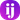Home /

# 🎄 Advent of Code Day 4 2019

I used Quokka.js to solve this problem. It lets you get the values of your code directly inline.

tldr; We have to find the count of numbers within a range that follow these rules:

1. It is a six-digit number.
2. The value is within the range given in your puzzle input.
3. Two adjacent digits are the same (like 22 in 122345).
4. Going from left to right, the digits never decrease; they only ever increase or stay the same (like 111123 or 135679).

The first thing I needed to do was create an array with the range of numbers the problem gave me.

`.css-1baulvz{display:inline-block;}const range = (j, k) => {  return Array(k - j + 1)    .fill()    .map((_, index) => j + index)}`

`Array(k - j + 1)` will create an array with the length `k - j + 1` passed in. `.fill()` makes each entry in the array `undefined` so that we can iterate over it. `.map((_, idx) => j + idx)` fills each element in the array starting with the `j` value and incrementing by the index.

`const OUR_RANGE = range(10, 15)// [10, 11, 12, 13, 14, 15]`

Next, we need to be able to iterate over any given number and check out rules against that number.

One way to create an array of numbers out of a single number is to convert it to a string, split that string and then map over the array and convert each back to a number.

`const digits = (n) => n.toString().split('').map(Number)`

My thought here was that we will create 1 reduce function per rule and filter the range down to the numbers that match each rule.

I am going to start with rule 4 because that will reduce the most amount of numbers with a single rule.

We want to use `Array.reduce` because we want to take the array of numbers and reduce it down to a `true` or `false` value.

`const numberIncreasesLeftToRight = (n) => {  return digits(n).reduce((acc, curr, index, array) => {}, true)}`

We start with `true` so that we can return early if we find a false value.

`const numberIncreasesLeftToRight = (n) => {  return digits(n).reduce((acc, curr, index, array) => {    if (!acc) {      return false    }  }, true)}`

Next, we need to check if the current number is less than or equal to the next number in the array. We return this value as the accumulator because if it ever returns false, we will return early.

`const numberIncreasesLeftToRight = (n) => {  return digits(n).reduce((acc, curr, index, array) => {    if (!acc) {      return false    }    return curr <= array[index + 1]  }, true)}`

This code has a bug in it. When we get to the last element in the array, `array[index + 1]` will return `undefined` causing `false` to be returned.

`const numberIncreasesLeftToRight = (n) => {  return digits(n).reduce((acc, curr, index, array) => {    if (!acc) {      return false    }    if (index + 1 === array.length) {      return acc    }    return curr <= array[index + 1]  }, true)}`

Next we will create a method for finding pairs in the number. This method will `reduce` the array of numbers just like `numberIncreasesLeftToRight` did. We start with a `false` value because we want to return early if we find a pair in the number.

`const numberHasPair = (n) => {  return digits(n).reduce((acc, curr, index, array) => {    if (acc) {      return true    }  }, false)}`

Next we want to check if the next number in the array is the same as the current number.

`const numberHasPair = (n) => {  return digits(n).reduce((acc, curr, index, array) => {    if (acc) {      return true    }    return curr === array[index + 1]  }, false)}`

And just like the last rule, we want to return the accumulated value if we get to the last number in the array.

`const numberHasPair = (n) => {  return digits(n).reduce((acc, curr, index, array) => {    if (acc) {      return true    }    if (index + 1 === array.length) {      return acc    }    return curr === array[index + 1]  }, false)}`

Now, putting everything together, we can find all the possible numbers given those 4 rules:

`const MIN_RANGE = 356261const MAX_RANGE = 846303const INPUT_RANGE = range(MIN_RANGE, MAX_RANGE)const result = INPUT_RANGE.filter(numberIncreasesLeftToRight).filter(  numberHasPair)`

If you filter the other way, you code will take about 25% longer to do so. I found this out by using quokka's `//?.` comment which tells you how long a line took to execute.